Courses

# RD Sharma Solutions - Ex-18.3, Symmetry, Class 7, Math Class 7 Notes | EduRev

## RD Sharma Solutions for Class 7 Mathematics

Created by: Abhishek Kapoor

## Class 7 : RD Sharma Solutions - Ex-18.3, Symmetry, Class 7, Math Class 7 Notes | EduRev

The document RD Sharma Solutions - Ex-18.3, Symmetry, Class 7, Math Class 7 Notes | EduRev is a part of the Class 7 Course RD Sharma Solutions for Class 7 Mathematics.
All you need of Class 7 at this link: Class 7

#### Question 1:

Give the order of rotational symmetry for each of the following figures when rotated about the marked point (x):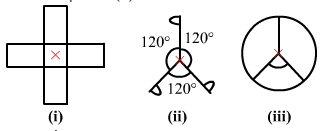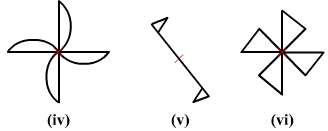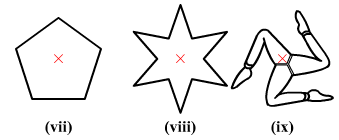(i) The given figure has its rotational symmetry as 4.
(ii) The given figure has its rotational symmetry as 3.
(iii) The given figure has its rotational symmetry as 3.
(iv) The given figure has its rotational symmetry as 4.
(v) The given figure has its rotational symmetry as 2.
(vi) The given figure has its rotational symmetry as 4.
(vii) The given figure has its rotational symmetry as 5.
(viii) The given figure has its rotational symmetry as 6.
(ix) The given figure has its rotational symmetry as 3.

#### Question 2:

Name any two figures that have both line symmetry and rotational symmetry.

An equilateral triangle and a square have both lines of symmetry and rotational symmetry.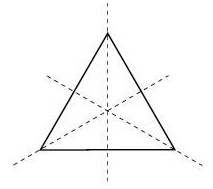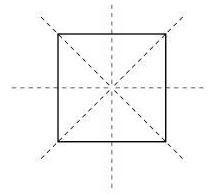Equilateral Triangle                                            Square

#### Question 3:

Give an example of a figure that has a line of symmetry but does not have rotational symmetry.

A semicircle and an isosceles triangle have a line of symmetry but do not have rotational symmetry.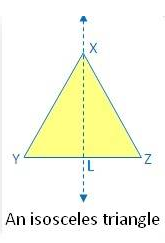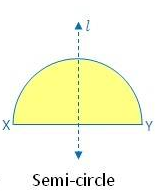#### Question 4:

Give an example of a geometrical figure which has neither a line of symmetry nor a rotational symmetry.

A scalene triangle has neither a line of symmetry nor a rotational symmetry.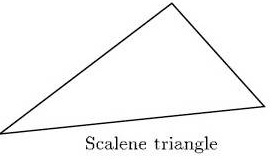#### Question 5:

Give an example of a letter of the English alphabet which has (i) no line of symmetry and (ii) rotational symmetry of order 2.

(i) The letter of the English alphabet which has no line of symmetry is Z.
(ii) The letter of the English alphabet which has rotational symmetry of order 2 is N.

#### Question 6:

What is the line of symmetry of a semi-circle? Does it have rotational symmetry?

A semicircle (half of a circle) has only one line of symmetry .
In the figure, there is one line of symmetry. The figure is symmetric along the perpendicular bisector l of the diameter XY.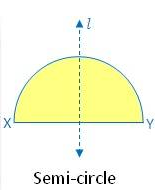A semi-circle does not have any rotational symmetry.

#### Question 7:

Draw, whenever possible, a rough sketch of
(i) a triangle with both line and rotational symmetries.
(ii) a triangle with only line symmetry and no rotational symmetry.
(iii) a quadrilateral with a rotational symmetry but not a line of symmetry.
(iv) a quadrilateral with line symmetry but not a rotational symmetry.

(i) An equilateral triangle has 3 lines of symmetry and a rotational symmetry of order 3.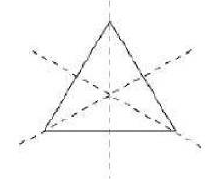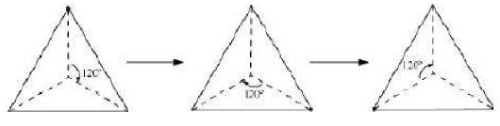(ii) An isosceles triangle has only 1 line of symmetry and no rotational symmetry.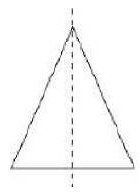(iii) A parallelogram is a quadrilateral which has no line of symmetry but a rotational symmetry of order 2.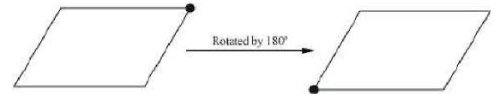(iv) A kite is a quadrilateral which has only one line of symmetry and no rotational symmetry.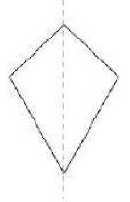#### Question 8:

Fill in the blanks: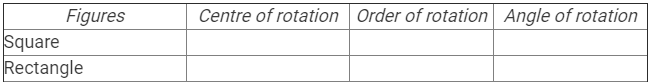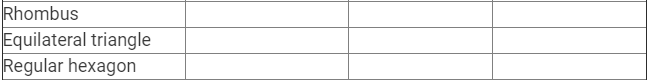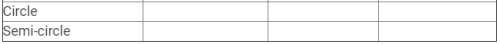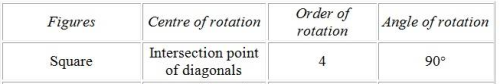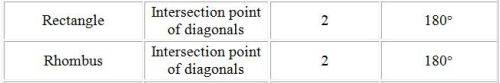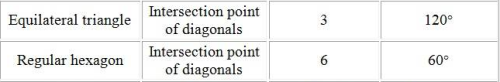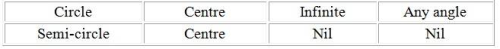#### Question 9:

Fill in the blanks: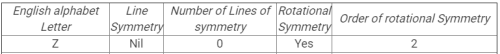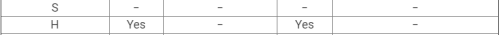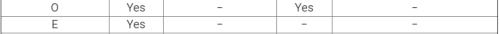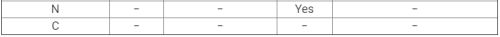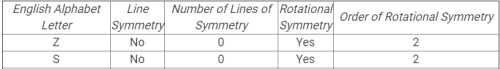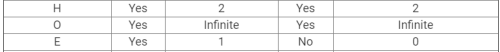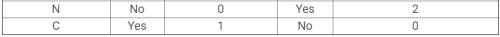97 docs

,

,

,

,

,

,

,

,

,

,

,

,

,

,

,

,

,

,

,

,

,

,

,

,

,

,

,

,

,

,

;